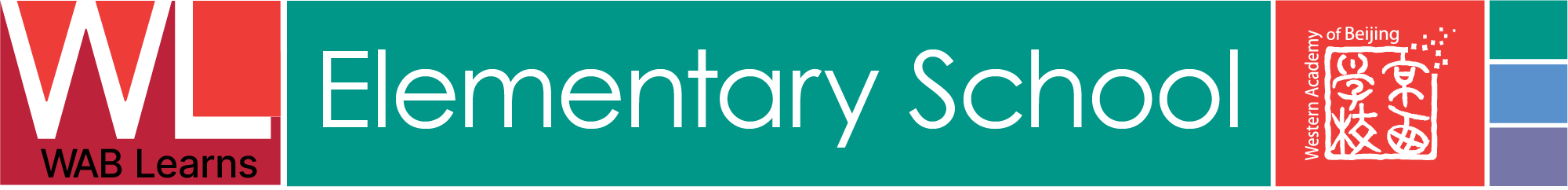# ES Mathematics: JG1

## UNITS

Unit:
• Number Names and Counting
Essential Questions:
• How can counting help us learn number names?
• How can we use objects to count and recognize numbers?
• How do numbers help us understand more about the world around us?
• How do patterns help us understand and count numbers?
• How can we use counting and numbers to solve simple math problems?
• What strategies can we use to remember and recognize numbers?
• How can we use counting and numbers to predict what will happen next?
• What is the connection between counting and learning number names?
• How do numbers help us understand the importance of order?
• How is counting related to solving real-world problems?
Unit:
• Number Sense
Essential Questions:
• How can numbers be used to represent and compare different quantities?
• How can numbers be manipulated to complete mathematical operations?
• How can objects be used to represent and compare different quantities?
• How can images be used to represent and compare different quantities?
• What are the best strategies for counting, sorting, and ordering objects?
• How can students recognize and name numbers up to 20?
• How can students identify and create patterns in numbers?
• How can students use problem solving strategies to solve math problems?
• What strategies can be used to solve math word problems?
• How can students use numbers to estimate and measure?
Unit:
• Fractions
Essential Questions:
• What is a fraction?
• How do fractions help us understand the world around us?
• How do fractions help us solve math problems?
• How can we represent fractions using objects or pictures?
• How can we compare fractions?
• How do fractions help us understand the concept of sharing?
• How can real-world objects be divided into fractions?
Unit:
• 2D and 3D Shapes
Essential Questions:
• How can basic shapes form more complex objects?
• How can shapes be used to solve problems?
• How do 2D and 3D shapes differ?
• What properties can you identify in each shape?
• How can you measure the area and perimeter of shapes?
• What patterns can be seen when exploring 2D and 3D shapes?
• How can 2D and 3D shapes be combined to create new objects?
• How can shapes be used to represent information?
Unit:
• Data Collection
Essential Questions:
• What can we learn from collecting data?
• How can data be organized and presented?
• How do we tell if the data is accurate?
• How can we use data to draw conclusions?
• What strategies can we use to collect data?
• How can we use data to compare and contrast different groups or objects?
• How can we use data to solve problems?
• How can we use data to make decisions?
Unit:
• Problem Solving and Communicating
Essential Questions:
• What strategies can we use to solve math problems?
• How can we use language to explain our thoughts while solving math problems?
• How can we share our problem-solving strategies with others?
• What happens when we collaborate to solve math problems?
• How can we use patterns to solve math problems?
• What challenges do we face when trying to solve math problems?
• How can we encourage and support one another when solving math problems?
• How does communication help us solve math problems?
Unit:
Essential Questions:
• How are numbers connected to each other through a variety of relationships?
• What does it mean to add and subtract numbers?
• How can we solve addition and subtraction problems?
• What strategies can we use to help us remember how to add and subtract?
• How can we use objects to help us learn addition and subtraction?
• How can we use pictures to help us solve addition and subtraction problems?
• What are the differences between addition and subtraction?
• How can we use our knowledge of addition and subtraction to solve real-world problems?
Unit:
• Place Value
Essential Questions:
• How can place value help us understand how quantities are connected?
• How can we represent numbers using digits?
• What is the value of each digit in a number?
• What is the base-10 system and how is it used?
• How can we use place value to compare and order numbers?
Unit:
• Attributes of Objects/Events
Essential Questions:
• What is an attribute?
• What are some attributes of different objects or events?
• How can we use attributes to help us distinguish between objects and events?
• How can we use attributes to compare and sort objects and events?
• How do different people identify attributes of objects and events?
• What is the importance of recognizing attributes of objects and events?
• How can recognizing attributes help us in solving problems?
Unit:
• Problem Solving and Reasoning
Essential Questions:
• What does it look like to solve a problem?
• How can we use our math skills to solve problems?
• How can we develop our problem solving skills?
• How do different strategies help us solve problems?
• What steps do we take to reason and explain our thinking?
• How do we use our counting skills to solve problems?
• How can we use visual representations to solve problems?
• What does it mean to think logically when solving a problem?
• How does understanding patterns help us solve problems?
• How can problems help us understand math concepts?